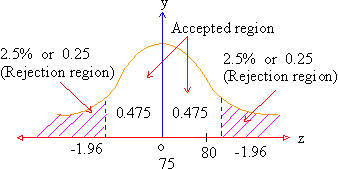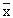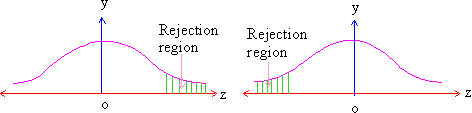Support the Monkey! Tell All your Friends and TeachersHome MonkeyNotes Printable Notes Digital Library Study Guides Study Smart Parents Tips College Planning Test Prep Fun Zone Help / FAQ How to Cite Request a New Title

8.16 Two tailed and One tailed Tests

While testing a hypothesis, we often talk of two-tailed tests and one-tailed tests. In the previous tests the critical region lay along both the tails of the distributions. That is, we did not want sample statistic ( say mean ) to be away from the population parameter (say mean) in either direction. The test for such a hypothesis is non-directional or two-sided or two-tailed. A two-tailed test of hypothesis will reject the null hypothesis Ho, if the sample statistic is significantly higher than or lower than the hypothesized population parameter. Thus in two-tailed test the rejection (critical) region is located in both the tails.

For example, suppose you suspect that a particular 6th graders performance on a test in Mathematics is not a true representative of the students who have appeared. The national mean score in this test was found to be 75. The alternative (or research) hypothesis is :

Ha : m ¹ 75 while the null hypothesis is : Ho : m = 75.

Now our pre-determined probability level is 95% i.e. 5% level of significance for this test. Both tests have the rejection (or critical) region of 5% i.e. 0.05. Now this rejection region is divided between both the tails of the distribution ( see figure 1 ) i.e. 2.5% or 0.25 in the upper tail and 2.5% or 0.25 in the upper tail and 2.5% or 0.25 in the lower tail since your hypothesis gives only a difference and not a direction. You will reject the null hypothesis on the basis that the sample mean falls into the area beyond 1.96 S.E. Otherwise if it falls into area 0.475 corresponds to 1.96 S.E. you can accept the null hypothesis.Suppose you want to reduce the risk of committing a Type I error, reduce the size of the rejection region. If the hypothesis is treated at 1% i.e. 0.01 level of significance. If we consult the table of areas under the normal curve (table - 2), we find the acceptance region of 0.495 ( (one half of .99) is equal to 2.58 S.E. from mH i.e. z-score = 0.You will still reject the null hypothesis of no difference, if the class sample is either much higher or much lower than our population mean of 75.Your browser does not support the IFRAME tag.

As distinguished from the two-tailed test, we can apply a directional - one sided i.e. one-tailed test also because in some cases it is necessary to guard against only small values of(i.e. sample mean). One-tailed test is so called because the rejection region will be located in only one-tail, which may either be on the upper or the lower side of the distribution depending upon the alternative ( Ha ) hypothesis formula. For example, we want to test a hypothesis that the average income per household is greater than \$ 5000 against the alternative hypothesis that the income is \$ 1000 or more. We will place all a risk on the upper-side of the theoretical sampling distribution and the test will be one-tailed. On the other hand, if we are testing that the average income per household is \$ 5000 against Ha that the income is less than \$ 5000 or less, the a risk is on the lower side of the distribution and the test will be one sided.Summing up, if the population as specified mean say mo then the null hypothesis would be Ho : m = mo and alternative (researchers) hypothesis could be :

1) Ha : m ¹ mo ( i.e. m > mo or m < mo )

2) Ha : m > mo

3) Ha : m < mo

Index

8.1 Population
8.2 Sample
8.3 Parameters and Statistic
8.4 Sampling Distribution
8.5 Sampling Error
8.6 Central Limit Theorem
8.7 Critical Region
8.8 Testing of Hypothesis
8.9 Errors in Tesitng of Hypothesis
8.10 Power o a Hypothesis Test
8.11 Sampling of Variables
8.12 Sampling of Attributes
8.13 Estimation
8.14 Testing the Difference Between Means
8.15 Test for Difference Between Proportions
8.16 Two Tailed and one Tailed Tests
8.17 Test of Significance for Small Samples
8.18 Students t-distribution
8.19 Distribution of 't' for Comparison of Two Samples Means Independent Samples
8.20 Testing Difference Between Mens of Two Samples Dependent Samples or Matched Paired Observations
8.21 Chi-Square
8.22 Sampling Theory of Correlation
8.23 Sampling Theory of Regression

Chapter 1Search: All Products Books Popular Music Classical Music Video DVD Toys & Games Electronics Software Tools & Hardware Outdoor Living Kitchen & Housewares Camera & Photo Cell Phones Keywords: